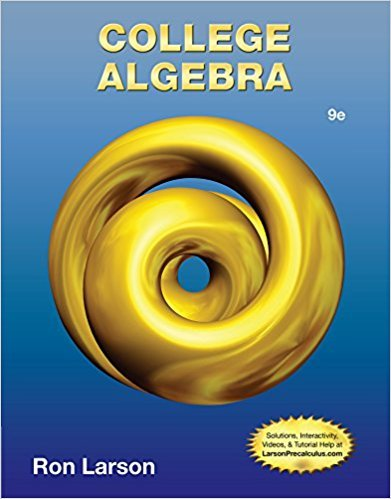×
×

# In Exercises 6974, find the center and radius of theISBN: 9781133963028 204

## Solution for problem 1.1.69 Chapter 1

College Algebra | 9th Edition

• Textbook Solutions
• 2901 Step-by-step solutions solved by professors and subject experts
• Get 24/7 help from StudySoup virtual teaching assistantsCollege Algebra | 9th Edition

4 5 0 319 Reviews
31
5
Problem 1.1.69

In Exercises 6974, find the center and radius of the circle. Then sketch the graph of the circle. x 2 36 2 y 2 25

Step-by-Step Solution:
Step 1 of 3

9/12: Quantifiers: 1. Universal: x ∈ D, P(x) a. For all x element of domain, such that P(x) i. P(x) is predicate 2. Existential: x ∈ D, Q(x) a. There exists an x element of domain, such that Q(x) i. Q(x) is quality 2 3. Ex. 1: x ∈ R, x...

Step 2 of 3

Step 3 of 3

##### ISBN: 9781133963028

The full step-by-step solution to problem: 1.1.69 from chapter: 1 was answered by , our top Math solution expert on 01/02/18, 09:21PM. This textbook survival guide was created for the textbook: College Algebra, edition: 9. The answer to “In Exercises 6974, find the center and radius of the circle. Then sketch the graph of the circle. x 2 36 2 y 2 25” is broken down into a number of easy to follow steps, and 25 words. College Algebra was written by and is associated to the ISBN: 9781133963028. This full solution covers the following key subjects: . This expansive textbook survival guide covers 9 chapters, and 5750 solutions. Since the solution to 1.1.69 from 1 chapter was answered, more than 225 students have viewed the full step-by-step answer.

Unlock Textbook Solution